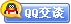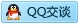##用户名 Email 自动登录 找回密码 密码 会员注册
 VIP会员，3年作业免费下 ！ 奥鹏作业，奥鹏毕业论文检测 新手作业下载教程，充值问题 没有找到答案，请在此处留言！ 2020年07月最新全国统考资料 投诉建议，加盟合作！奥鹏课程积分软件(ver:3.1)

# 2012年春季学期《C语言》在线考试(适用于2012年7月份考试)发表于 2012-6-25 17:28:48 | 显示全部楼层 |阅读模式一、单选题（共 10 道试题，共 20 分。）V 1.  设有定义：int x=12,n=5; 则表达式 x%=(n%2) 的值为 A. 0 B. 1 C. 2 D. 3       满分：2  分 2.  假设已定义 char c= "test"； int i； 则下面的输出函数调用中错误的是 A. printf("%s",c)； B. for(i=0；i<8；i++) printf("%c",c[i])； C. puts(c) D. for(i=0；i<8；i++) puts(c[i])；       满分：2  分 3.  当对两个字符串进行比较时，应该使用的函数是 A. strcat B. strcmp C. strcpy D. strlen       满分：2  分 4.  下列数组定义及赋值不正确的是 A. int a={0,1,2,3,4,5,6,7,8,9}； B. int a[ ]={0,1,2,3,4,5,6,7,8,9}； C. int a= {0,1,2,3,4,5,6,7,8,9}； D. int a[ ]= {0,1,2,3,4,5,6,7,8,9}；       满分：2  分 5.  若有以下定义：char s= "programming"，*ps=s；则不能代表字符o的表达式是 A. ps+2 B. s C. ps D. ps+=2,*ps       满分：2  分 6.   已定义以下函数: fun(int *p)                 { return *p; } 该函数的返回值是 A. 不确定的值 B. 形参p所指存储单元中的值 C. 形参p中存放的值 D. 形参p的地址值       满分：2  分 7.  下列变量的定义及赋值正确的是 A. int i=0;j; B. char c=”A”; C. double x,y,z; x=y=z=100; D. float a=b=100;       满分：2  分 8.  下列循环语句中，不能够正常结束的是 A.   int i=0; for(;i<100;i++); B. int i=0; for(;;){if(i++>100)break;} C. int i=0,j=0; while(i<=j)j++; D. int i=0,j=0; do{i+=j;i++;j++;}while(i void main()      { int a,*p,*s,i;       for(i=0;i<10;i++)  scanf(%d”,a+i);       for(p=a,s=a;p-a<10;p++)  if(*p>*s)s=p;        printf(“max=%d,index=%d\n”,*s, s-a); } 简述上列程序完成的功能：       满分：5  分 2.   读程序写出程序执行结果 #include int fun(int x)     {  return(x>0 ? x : -x);  } void main() {  int a=-5;     printf(“%d, %d\n”, a, fun(a+10)); }       满分：5  分 3.   读程序写出程序执行结果 #include void main()    {  int x=2,y=6;       int j;       for(j=1 ; y>0 ; y -- )  j=j*x;       printf(“j=%d\n”,j); }       满分：5  分 4.   读程序写出程序执行结果 #include void main() { int i=0,a=0; while( i<20 ) {  for(;;)       if((i%10)= =0) break;        else i- -;        i+=11;        a+=i; } printf("%d\n",a); }       满分：5  分 5.   读程序写出程序执行结果 #include void main() { char  x[]="language"; char *ptr=x; while(*ptr)   {  printf("%c\n",*ptr-32);      ptr++;   } }       满分：5  分 6.   读程序写出程序执行结果 #include void main() {  char x[]="123456789",*p=x ;       int i=0;    while(*p)     {  if(i%2==0) *p='*’;        p++; i++;     }   puts(x); }       满分：5  分 7.   读程序写出程序执行结果 #include void main ( ) {  char  a= "abcXYZ", c ;    int  i,  j;    j= strlen(a)-1 ;    for (i=0;  j>i;  i++，j--)        {  c=*(a+i); *(a+i)=*(a+j); *(a+j)=c;  }          puts(a);    }       满分：5  分 8.   读程序写出程序执行结果 #include int fib(int g) { switch(g)    {  case 0: return 0;       case 1:       case 2: return 1;    }    return -1; } void main() {  printf(“%d\n”, fib(5)) ; }       满分：5  分 9.   读程序写出程序执行结果 #include void main() { int x=26587,x1,r,sum=0; x1=x; while(x>0) { r=x%10; sum+=r; x/=10; } printf( "Sum of the digits in %d is %d", x1,sum); }       满分：5  分 10.   读程序写出程序执行结果 #include void main() { int y=-1 ； do {  y++； }while(y--)；        printf("%d\n"，y--)；      }       满分：5  分 三、简答题（共 3 道试题，共 30 分。）V 1.  编程：任意输入100个整数，分别统计其中的正数、负数和零的个数。（备注：建议学员在做编程序题目时，事先在记事本或word文档中编写，然后拷贝至答题区域即可。）       满分：10  分 2.   编程题 备注：建议学员在做编程序题目时，事先在记事本或word文档中编写，然后拷贝至答题区域即可。       满分：10  分 3.   编程：写一个判断素数的函数。要求在主函数中输入一个正整数以及打印函数调用后返回的相应的判断信息。（备注：建议学员在做编程序题目时，事先在记事本或word文档中编写，然后拷贝至答题区域即可。）       满分：10  分
 本版积分规则 回帖并转播 回帖后跳转到最后一页客服一客服二客服三客服四微信客服扫一扫